## Applications of electromagnetic induction

7-22-99

Electromagnetic induction is an incredibly useful phenomenon with a wide variety of applications. Induction is used in power generation and power transmission, and it's worth taking a look at how that's done. There are other effects with some interesting applications to consider, too, such as eddy currents.

### Eddy currents

An eddy current is a swirling current set up in a conductor in response to a changing magnetic field. By Lenząs law, the current swirls in such a way as to create a magnetic field opposing the change; to do this in a conductor, electrons swirl in a plane perpendicular to the magnetic field.

Because of the tendency of eddy currents to oppose, eddy currents cause energy to be lost. More accurately, eddy currents transform more useful forms of energy, such as kinetic energy, into heat, which is generally much less useful. In many applications the loss of useful energy is not particularly desirable, but there are some practical applications. One is in the brakes of some trains. During braking, the metal wheels are exposed to a magnetic field from an electromagnet, generating eddy currents in the wheels. The magnetic interaction between the applied field and the eddy currents acts to slow the wheels down. The faster the wheels are spinning, the stronger the effect, meaning that as the train slows the braking force is reduced, producing a smooth stopping motion.

### An electric generator

A electric motor is a device for transforming electrical energy into mechanical energy; an electric generator does the reverse, using mechanical energy to generate electricity. At the heart of both motors and generators is a wire coil in a magnetic field. In fact, the same device can be used as a motor or a generator.

When the device is used as a motor, a current is passed through the coil. The interaction of the magnetic field with the current causes the coil to spin. To use the device as a generator, the coil can be spun, inducing a current in the coil.

An AC (alternating current) generator utilizes Faraday's law of induction, spinning a coil at a constant rate in a magnetic field to induce an oscillating emf. The coil area and the magnetic field are kept constant, so, by Faraday's law, the induced emf is given by: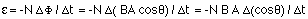If the loop spins at a constant rate,. Using calculus, and taking the derivative of the cosine to get a sine (as well as bringing out a factor of), it's easy to show that the emf can be expressed as: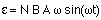The combination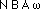represents the maximum value of the generated voltage (i.e., emf) and can be shortened to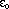. This reduces the expression for the emf to: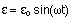In other words, a coil of wire spun in a magnetic field at a constant rate will produce AC electricity. In North America, AC electricity from a wall socket has a frequency of 60 Hz.

A coil turning in a magnetic field can also be used to generate DC power. A DC generator uses the same kind of split-ring commutator used in a DC motor. Unlike the AC generator, the polarity of the voltage generated by a DC generator is always the same. In a very simple DC generator with a single rotating loop, the voltage level would constantly fluctuate. The voltage from many loops (out of synch with each other) is usually added together to obtain a relatively steady voltage.

Rather than using a spinning coil in a constant magnetic field, another way to utilize electromagnetic induction is to keep the coil stationary and to spin permanent magnets (providing the magnetic field and flux) around the coil. A good example of this is the way power is generated, such as at a hydro-electric power plant. The energy of falling water is used to spin permanent magnets around a fixed loop, producing AC power.

### Back EMF in electric motors

You may have noticed that when something like a refrigerator or an air conditioner first turns on in your house, the lights dim momentarily. This is because of the large current required to get the motor inside these machines up to operating speed. When the motors are turning, much less current is necessary to keep them turning.

One way to analyze this is to realize that a spinning motor also acts like a generator. A motor has coils turning inside magnetic fields, and a coil turning inside a magnetic field induces an emf. This emf, known as the back emf, acts against the applied voltage that's causing the motor to spin in the first place, and reduces the current flowing through the coils. At operating speed, enough current flows to overcome any losses due to friction and to provide the necessary energy required for the motor to do work. This is generally much less current than is required to get the motor spinning in the first place.

If the applied voltage is V, then the initial current flowing through a motor with coils of resistance R is I = V / R. When the motor is spinning and generating a back emf, the current is reduced: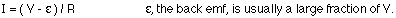### Mutual inductance

Faraday's law tells us that a changing magnetic flux will induce an emf in a coil. The induced emf for a coil with N loops is: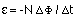Picture two coils next to each other, end to end. If the first coil has a current going through it,a magnetic field will be produced, and a magnetic flux will pass through the second coil. Changing the current in the first coil changes the flux through the second, inducing an emf in the second coil. This is known as mutual inductance, inducing an emf in one coil by changing the current through another. The induced emf is proportional to the change in flux,which is proportional to the change in current in the first coil. The induced emf can thus be written as: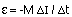The constant M is the mutual inductance, which depends on various factors, including the area and number of turns in coil 2, the distance between the two coils (the further apart, the less flux passes through coil 2), the relative orientation of the two coils, the number of turns / unit length in the first coil (because that's what the magnetic field produced by the first coil depends on), and whether the two coils have cores made from ferromagentic material. In other words, M is rather complicated. What's far more important in the equation above is that the emf induced in the second coil is proportional to the change in current in the first.

This effect can be put to practical use. One way to use it is in a transformer, which we'll discuss below. Another is to use it in an ammeter. Conventional ammeters are incorporated directly into circuits, but ammeters don't have to be placed in the current path for alternating current. If a loop connected to a meter is placed around a wire with an AC current in it, an emf will be induced in the loop because of the changing field from the wire, and that will produce a current in the loop, and meter, proportional to the current in the wire.

### Self inductance

Coils can also induce emf's in themselves. If a changing current is passed through a coil, a changing magnetic field will be produced, inducing an emf in the coil. Again, this emf is given by:As with mutual inductance, the induced emf is proportional to the change in current. The induced emf can be written as: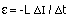The constant L is known as the inductance of the coil. It depends on the coil geometry, as well as on whether the coil has a core of ferromagnetic material.

We've already discussed resistors and capacitors as circuit elements. Inductors, which are simply wire coils, often with ferromagnetic cores, are another kind of circuit element. One of the main differences between these is what happens to electrical energy in them. Resistors dissipate electrical energy in the form of heat; capacitors store the energy in an electric field between the capacitor plates; and inductors store the energy in the magnetic field in the coil. The energy stored in an inductor is: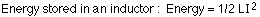In general, the energy density (energy per unit volume) in a magnetic field is: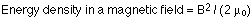### Transformers

Electricity is often generated a long way from where it is used, and is transmitted long distances through power lines. Although the resistance of a short length of power line is relatively low, over a long distance the resistance can become substantial. A power line of resistance R causes a power loss of I2R ; this is wasted as heat. By reducing the current, therefore, the I2R losses can be minimized.

At the generating station, the power generated is given by P = VI. To reduce the current while keeping the power constant, the voltage can be increased. Using AC power, and Faraday's law of induction, there is a very simple way to increase voltage and decrease current (or vice versa), and that is to use a transformer. A transformer is made up of two coils, each with a different number of loops, linked by an iron core so the magnetic flux from one passes through the other. When the flux generated by one coil changes (as it does continually if the coil is connected to an AC power source), the flux passing through the other will change, inducing a voltage in the second coil. With AC power, the voltage induced in the second coil will also be AC.

In a standard transformer, the two coils are usually wrapped around the same iron core, ensuring that the magnetic flux is the same through both coils. The coil that provides the flux (i.e., the coil connected to the AC power source) is known as the primary coil, while the coil in which voltage is induced is known as the secondary coil. If the primary coil sets up a changing flux, the voltage in the secondary coil depends on the number of turns in the secondary: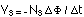Similarly, the relationship for the primary coil is: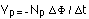Combining these gives the relationship between the primary and secondary voltage: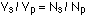Energy (or, equivalently, power) has to be conserved, so: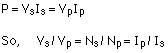If a transformer takes a high primary voltage and converts it to a low secondary voltage, the current in the secondary will be higher than that in the primary to compensate (and vice versa). A transformer in which the voltage is higher in the primary than the secondary (i.e., more turns in the primary than the secondary) is known as a step-down transformer. A transformer in which the secondary has more turns (and, therefore, higher voltage) is known as a step-up transformer.

Power companies use step-up transformers to boost the voltage to hundreds of kV before it is transmitted down a power line, reducing the current and minimizing the power lost in transmission lines. Step-down transformers are used at the other end, to decrease the voltage to the 120 or 240 V used in household circuits.

Transformers require a varying flux to work. They are therefore perfect for AC power, but do not work at all for DC power, which would keep the flux constant. The ease with which voltage and current can be tranformed in an AC circuit is a large part of the reason AC power, rather than DC, is distributed by the power companies.

Although transformers dramatically reduce the energy lost to I2R heating in power line, they don't give something for nothing. Transformers will also dissipate some energy, in the form of:

1. flux leakage - not all the magnetic flux from the primary passes through the secondary
2. self-induction - the opposition of the coils to a changing flux in them
3. heating losses in the coils of the transformer
4. eddy currents

In the iron core of a transformer, electrons would swirl in cross-sectional planes. This current would heat up the transformer, wasting power as heat. To minimize power losses due to eddy currents, the iron core is usually made up of thin laminated slices, rather than one solid piece. Current is then confined within each laminated piece, significantly reducing the swirling tendency as well as the losses by heating.